# Getting Started with canvasXpress in R

## Examples

### Scatter 3D Plot

y <- read.table("http://www.canvasxpress.org/data/cX-irist-dat.txt", header=TRUE, sep="\t",
quote="", row.names=1, fill=TRUE, check.names=FALSE, stringsAsFactors=FALSE)
quote="", row.names=1, fill=TRUE, check.names=FALSE, stringsAsFactors=FALSE)

canvasXpress(data      = y,
varAnnot  = z,
colorBy   = "Species",
ellipseBy = "Species",
graphType = "Scatter3D",
title     = "Iris Data Set",
xAxis     = list("Sepal.Length"),
yAxis     = list("Petal.Width"),
zAxis     = list("Petal.Length"))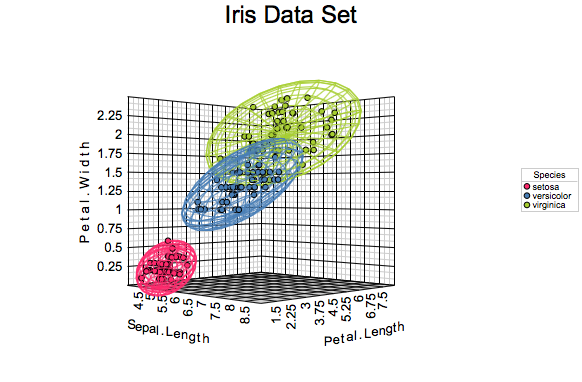Scatter3D

### Scatter 2D Matrix Plot

y <- read.table("http://www.canvasxpress.org/data/cX-irist-dat.txt", header=TRUE, sep="\t",
quote="", row.names=1, fill=TRUE, check.names=FALSE, stringsAsFactors=FALSE)
quote="", row.names=1, fill=TRUE, check.names=FALSE, stringsAsFactors=FALSE)

canvasXpress(data              = y,
varAnnot          = z,
graphType         = "Scatter2D",
scatterPlotMatrix = TRUE,
colorBy           = "Species",
showTransition    = TRUE)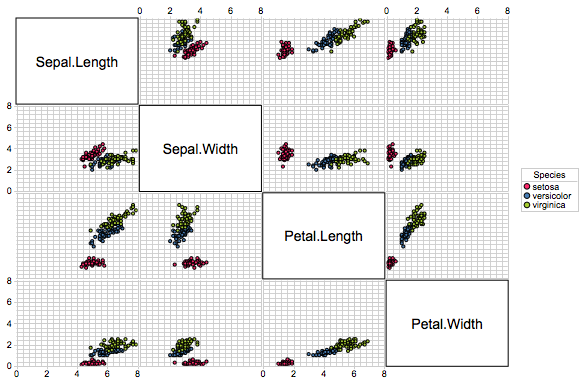Scatter2DMatrix

### Boxplot

y <- read.table("http://www.canvasxpress.org/data/cX-iris-dat.txt", header=TRUE, sep="\t",
quote="", row.names=1, fill=TRUE, check.names=FALSE, stringsAsFactors=FALSE)
quote="", row.names=1, fill=TRUE, check.names=FALSE, stringsAsFactors=FALSE)

canvasXpress(data              = y,
smpAnnot          = x,
graphType         = "Boxplot",
graphOrientation  = "vertical",
title             = "Iris flower data set",
smpTitle          = "Species",
smpLabelFontStyle = "italic",
smpLabelRotate    = 90,
xAxis2Show        = FALSE,
afterRender       = list(list("groupSamples", list("Species"))))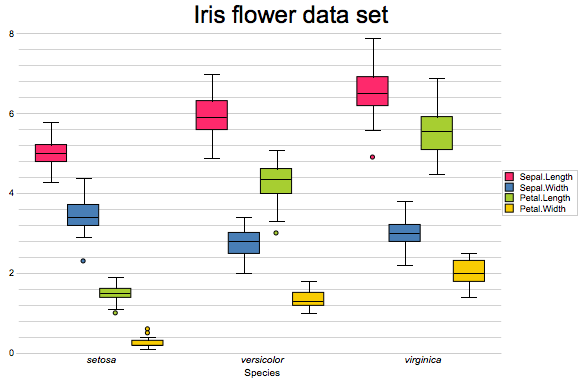Boxplot

### Heatmap

y  <- read.table("http://www.canvasxpress.org/data/cX-multidimensionalheatmap-dat.txt",
check.names=FALSE, stringsAsFactors=FALSE)
check.names=FALSE, stringsAsFactors=FALSE)
check.names=FALSE, stringsAsFactors=FALSE)
check.names=FALSE, stringsAsFactors=FALSE)
header=TRUE, sep= "\t", quote="", row.names=1, fill=TRUE,
check.names=FALSE, stringsAsFactors=FALSE)
header=TRUE, sep= "\t", quote="", row.names=1, fill=TRUE,
check.names=FALSE, stringsAsFactors=FALSE)

canvasXpress(data          = list(y = y, data2 = y2, data3 = y3, data4 = y4),
smpAnnot      = x,
varAnnot      = z,
graphType     = "Heatmap",
guides        = TRUE,
outlineBy     = "Outline",
outlineByData = "data2",
shapeBy       = "Shape",
shapeByData   = "data3",
sizeBy        = "Size",
sizeByData    = "data4")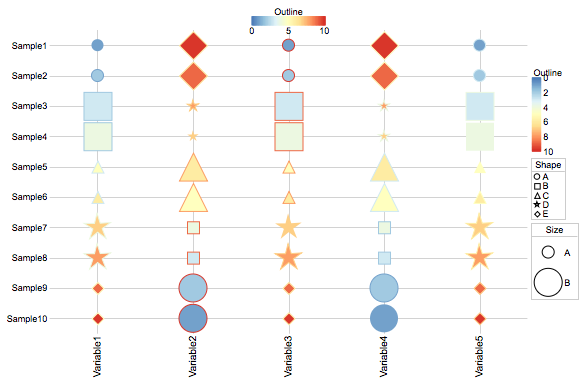Heatmap

### Four-way Venn Diagram

canvasXpress(vennData   = data.frame(AC=456, A=340, ABC=552, ABCD=148, BC=915,
ACD=298, BCD=613, B=562, CD=143, ABD=578,
graphType  = "Venn",
vennLegend = list(A="List 1", D="List 4", C="List 3", B="List 2"),
vennGroups = 4)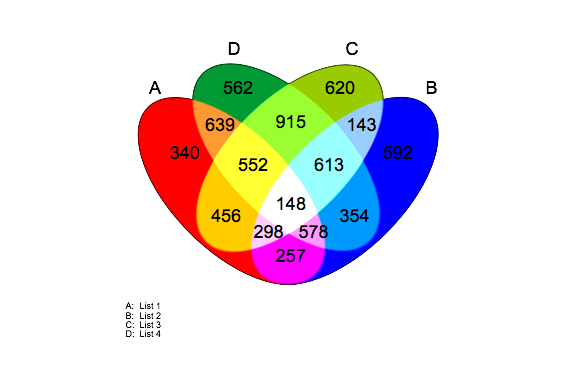Venn

#List example names
cxShinyExample(example = "example1")